• 贝塞尔曲线高阶匀速运动算法 HTML5/JS 实现,请关注我的博客谢谢
• 二次贝塞尔曲线通常以如下方式构建，给定二维平面上的固定点P0,P1,P2，用B(t)表示该条曲线 用一个动画来演示，可以更加清楚的表明这条曲线的构建...如何想要得到匀速贝塞尔曲线运动呢？比如我们在某款游戏中设...
转自：https://www.iteye.com/blog/as3-865587
二次贝塞尔曲线通常以如下方式构建，给定二维平面上的固定点P0,P1,P2，用B(t)表示该条曲线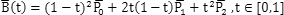用一个动画来演示，可以更加清楚的表明这条曲线的构建过程  如果t变量本身线形变化的话，这条贝塞尔曲线本身的生成过程是并不是匀速的，通常都是两头快中间慢。  如何想要得到匀速的贝塞尔曲线运动呢？比如我们在某款游戏中设计了一条贝塞尔曲线的路径，如何实现玩家匀速在这条路径上运动呢？  思考这个算法颇费了一番脑筋，其间还得到数学牛人Charlesgao的帮助，非常感谢他（比较糗的是，我问问题的时候就把其中的一个公式搞错了，见笑了-_-!)。  首先需要求得B(t)相对于t的速度公式s(t)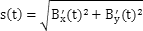为了简化公式，我们定义如下变量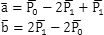计算出的s(t)可以表达为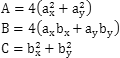其中A,B,C是根据P0,P1,P2计算出的常数根据这个公式，求得贝塞尔曲线的长度公式L(t)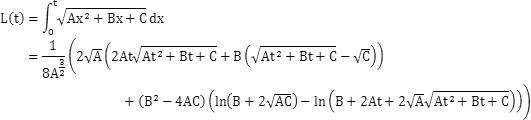设t就是能够使L实现匀速运动的自变量，那么显然L(t)=L(1.0)*t，即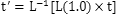由于L(t)函数非常复杂，直接求逆函数的表达式几乎不可能，还好我们可以知道它的导数为s(t)，在实际使用中，可以使用牛顿切线法求出近似解。其迭代算法可以表达为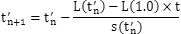我写了一个测试程序用于验证该算法，运算结果如下，可以看到，这条曲线已经是以匀速方式生成的了 ^_^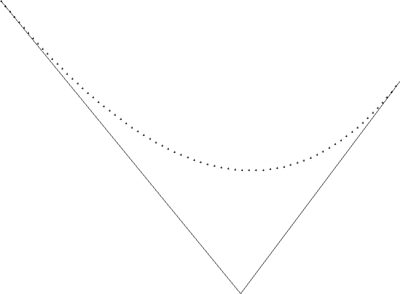完整的示例源代码:
#include <stdio.h>
#include <math.h>
#include <windows.h>

//三个控制点
POINT P0={50,50},P1={500,600},P2={800,200};

int ax = P0.x-2*P1.x+P2.x;
int ay = P0.y-2*P1.y+P2.y;
int bx = 2*P1.x-2*P0.x;
int by = 2*P1.y-2*P0.y;

double A = 4*(ax*ax+ay*ay);
double B = 4*(ax*bx+ay*by);
double C = bx*bx+by*by;

//曲线总长度
double total_length = 0.0;

//曲线分割的份数
const int STEP = 70;

//用于保存绘制点数据的数组
POINT pixels[STEP];

//-------------------------------------------------------------------------------------
//速度函数
/*
s(t_) = Sqrt[A*t*t+B*t+C]
*/
double s(double t)
{
return sqrt(A*t*t+B*t+C);
}

//-------------------------------------------------------------------------------------
//长度函数
/*

L(t) = Integrate[s[t], t]

L(t_) = ((2*Sqrt[A]*(2*A*t*Sqrt[C + t*(B + A*t)] + B*(-Sqrt[C] + Sqrt[C + t*(B + A*t)])) +
(B^2 - 4*A*C) (Log[B + 2*Sqrt[A]*Sqrt[C]] - Log[B + 2*A*t + 2 Sqrt[A]*Sqrt[C + t*(B + A*t)]]))
/(8* A^(3/2)));
*/
double L(double t)
{
double temp1 = sqrt(C+t*(B+A*t));
double temp2 = (2*A*t*temp1+B*(temp1-sqrt(C)));
double temp3 = log(B+2*sqrt(A)*sqrt(C));
double temp4 = log(B+2*A*t+2*sqrt(A)*temp1);
double temp5 = 2*sqrt(A)*temp2;
double temp6 = (B*B-4*A*C)*(temp3-temp4);

return (temp5+temp6)/(8*pow(A,1.5));
}

//-------------------------------------------------------------------------------------
//长度函数反函数，使用牛顿切线法求解
/*
X(n+1) = Xn - F(Xn)/F'(Xn)
*/
double InvertL(double t, double l)
{
double t1=t, t2;

do
{
t2 = t1 - (L(t1)-l)/s(t1);
if(abs(t1-t2)<0.000001) break;
t1=t2;
}while(true);
return t2;
}

//-------------------------------------------------------------------------------------
LRESULT CALLBACK _WndProc(HWND hWnd, UINT message, WPARAM wParam, LPARAM lParam)
{
switch (message)
{
case WM_TIMER:
{
static nIndex = 0;
if(nIndex>=0 && nIndex<=STEP)
{
double t = (double)nIndex/STEP;
//如果按照线形增长,此时对应的曲线长度
double l = t*total_length;
//根据L函数的反函数，求得l对应的t值
t = InvertL(t, l);

//根据贝塞尔曲线函数，求得取得此时的x,y坐标
double x = (1-t)*(1-t)*P0.x +2*(1-t)*t*P1.x + t*t*P2.x;
double y = (1-t)*(1-t)*P0.y +2*(1-t)*t*P1.y + t*t*P2.y;

//取整
pixels[nIndex].x = (int)(x+0.5);
pixels[nIndex].y = (int)(y+0.5);

nIndex++;
InvalidateRect(hWnd, 0, 0);
}
else
{
KillTimer(hWnd, 101);
}
}
break;
case WM_PAINT:
{
PAINTSTRUCT ps;
HDC hdc = BeginPaint(hWnd, &ps);
::MoveToEx(hdc, P0.x, P0.y, 0);
LineTo(hdc, P1.x, P1.y);
LineTo(hdc, P2.x, P2.y);

for(int i=0; i<STEP; i++)
{
const POINT &pt = pixels[i];
if(pt.x==0 && pt.y==0) break;

::MoveToEx(hdc, pt.x-2, pt.y, 0);
::LineTo(hdc, pt.x+2, pt.y);
::MoveToEx(hdc, pt.x, pt.y-2, 0);
::LineTo(hdc, pt.x, pt.y+2);
}
EndPaint(hWnd, &ps);
}
break;
case WM_DESTROY:
PostQuitMessage(0);
break;
default:
return DefWindowProc(hWnd, message, wParam, lParam);
}
return 0;
}

//-------------------------------------------------------------------------------------
int APIENTRY WinMain(HINSTANCE hInstance,
HINSTANCE hPrevInstance,
LPTSTR    lpCmdLine,
int       nCmdShow)
{
//注册窗口类
WNDCLASSEX wcex;
ZeroMemory(&wcex, sizeof(WNDCLASSEX));

wcex.cbSize = sizeof(WNDCLASSEX);
wcex.style			= CS_HREDRAW | CS_VREDRAW;
wcex.lpfnWndProc	= (WNDPROC)_WndProc;
wcex.hInstance		= hInstance;
wcex.hbrBackground	= (HBRUSH)(COLOR_WINDOW+1);
wcex.lpszClassName	= "BezierClass";
RegisterClassEx(&wcex);

//创建窗口
HWND hWnd = CreateWindow("BezierClass", "BezierDemo", WS_OVERLAPPEDWINDOW,
CW_USEDEFAULT, 0, CW_USEDEFAULT, 0, NULL, NULL, hInstance, NULL);
ShowWindow(hWnd, nCmdShow);
UpdateWindow(hWnd);

//计算总长度
total_length = L(1);

//清空绘制点数据
ZeroMemory(&pixels, sizeof(pixels));

//设定定时刷新计时器
SetTimer(hWnd, 101, 10, 0);

//消息循环
MSG msg;
while(GetMessage(&msg, NULL, 0, 0))
{
TranslateMessage(&msg);
DispatchMessage(&msg);
}

return (int) msg.wParam;
}


展开全文• cocos匀速贝塞尔曲线运动 const { ccclass, property } = cc._decorator; @ccclass export default class Expression extends cc.Component { // LIFE-CYCLE CALLBACKS: // onLoad() { } start() { cc....


cocos匀速贝塞尔曲线运动


const { ccclass, property } = cc._decorator;

@ccclass
export default class Expression extends cc.Component {

// LIFE-CYCLE CALLBACKS:

start() {
cc.tween(this as any)
.to(2, { factor: 1 })
.call(() => {
cc.Tween.stopAllByTarget(this.node);
this.node.destroy();
})
.start();
}

public get factor(): number {
return 0;
}

public set factor(value: number) {
this.node.x = (1 - value) * (1 - value) * 100 + 2 * value * (1 - value) * 300 + value * value * 100;
this.node.y = (1 - value) * (1 - value) * 100 + 2 * value * (1 - value) * 300 + value * value * 500;
}

// update (dt) {}
}


P0点是(100,100)，P1点是(300,300)，P2点是(100,500)。
展开全文cocos
• 二次贝塞尔曲线通常以如下方式构建，给定二维平面上的固定点P0,P1,P2，用B(t)表示该条曲线用一个动画来演示，可以更加清楚的表明这条曲线的构建...如何想要得到匀速贝塞尔曲线运动呢？比如我们在某款游戏中设计了...
二次贝塞尔曲线通常以如下方式构建，给定二维平面上的固定点P0,P1,P2，用B(t)表示该条曲线用一个动画来演示，可以更加清楚的表明这条曲线的构建过程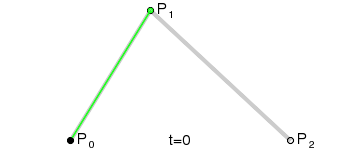如果t变量本身线形变化的话，这条贝塞尔曲线本身的生成过程是并不是匀速的，通常都是两头快中间慢。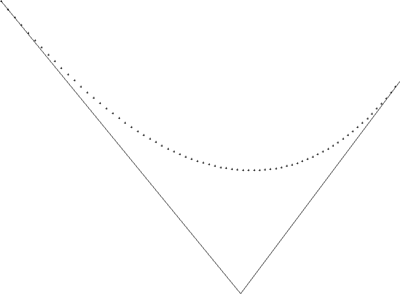如果t变量本身线形变化的话，这条贝塞尔曲线本身的生成过程是并不是匀速的，通常都是两头快中间慢。
如何想要得到匀速的贝塞尔曲线运动呢？比如我们在某款游戏中设计了一条贝塞尔曲线的路径，如何实现玩家匀速在这条路径上运动呢？思考这个算法颇费了一番脑筋，其间还得到数学牛人Charlesgao的帮助，非常感谢他（比较糗的是，我问问题的时候就把其中的一个公式搞错了，见笑了-_-!)。
首先需要求得B(t)相对于t的速度公式s(t)为了简化公式，我们定义如下变量：计算出的s(t)可以表达为：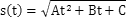其中A,B,C是根据P0,P1,P2计算出的常数：根据这个公式，求得贝塞尔曲线的长度公式L(t)：设t就是能够使L实现匀速运动的自变量，那么显然L(t)=L(1.0)*t，即：由于L(t)函数非常复杂，直接求逆函数的表达式几乎不可能，还好我们可以知道它的导数为s(t)，在实际使用中，可以使用牛顿切线法求出近似解。其迭代算法可以表达为：我写了一个测试程序用于验证该算法，运算结果如下，可以看到，这条曲线已经是以匀速方式生成的了 ^_^：[cpp]
view plain
copy

#include <stdio.h>    #include <math.h>    #include <windows.h>        //三个控制点    POINT P0={50,50},P1={500,600},P2={800,200};        int ax = P0.x-2*P1.x+P2.x;    int ay = P0.y-2*P1.y+P2.y;    int bx = 2*P1.x-2*P0.x;    int by = 2*P1.y-2*P0.y;        double A = 4*(ax*ax+ay*ay);    double B = 4*(ax*bx+ay*by);    double C = bx*bx+by*by;        //曲线总长度    double total_length = 0.0;        //曲线分割的份数    const int STEP = 70;        //用于保存绘制点数据的数组    POINT pixels[STEP];        //-------------------------------------------------------------------------------------    //速度函数    /*  s(t_) = Sqrt[A*t*t+B*t+C]  */    double s(double t)    {        return sqrt(A*t*t+B*t+C);    }        //-------------------------------------------------------------------------------------    //长度函数    /*    L(t) = Integrate[s[t], t]    L(t_) = ((2*Sqrt[A]*(2*A*t*Sqrt[C + t*(B + A*t)] + B*(-Sqrt[C] + Sqrt[C + t*(B + A*t)])) +               (B^2 - 4*A*C) (Log[B + 2*Sqrt[A]*Sqrt[C]] - Log[B + 2*A*t + 2 Sqrt[A]*Sqrt[C + t*(B + A*t)]]))                  /(8* A^(3/2)));  */    double L(double t)    {        double temp1 = sqrt(C+t*(B+A*t));        double temp2 = (2*A*t*temp1+B*(temp1-sqrt(C)));        double temp3 = log(B+2*sqrt(A)*sqrt(C));        double temp4 = log(B+2*A*t+2*sqrt(A)*temp1);        double temp5 = 2*sqrt(A)*temp2;        double temp6 = (B*B-4*A*C)*(temp3-temp4);                return (temp5+temp6)/(8*pow(A,1.5));    }        //-------------------------------------------------------------------------------------    //长度函数反函数，使用牛顿切线法求解    /*      X(n+1) = Xn - F(Xn)/F'(Xn)  */    double InvertL(double t, double l)    {        double t1=t, t2;                do        {            t2 = t1 - (L(t1)-l)/s(t1);            if(abs(t1-t2)<0.000001) break;            t1=t2;        }while(true);        return t2;    }        //-------------------------------------------------------------------------------------    LRESULT CALLBACK _WndProc(HWND hWnd, UINT message, WPARAM wParam, LPARAM lParam)    {        switch (message)         {        case WM_TIMER:            {                static nIndex = 0;                if(nIndex>=0 && nIndex<=STEP)                {                    double t = (double)nIndex/STEP;                    //如果按照线形增长,此时对应的曲线长度                    double l = t*total_length;                    //根据L函数的反函数，求得l对应的t值                    t = InvertL(t, l);                        //根据贝塞尔曲线函数，求得取得此时的x,y坐标                    double x = (1-t)*(1-t)*P0.x +2*(1-t)*t*P1.x + t*t*P2.x;                    double y = (1-t)*(1-t)*P0.y +2*(1-t)*t*P1.y + t*t*P2.y;                        //取整                    pixels[nIndex].x = (int)(x+0.5);                    pixels[nIndex].y = (int)(y+0.5);                        nIndex++;                    InvalidateRect(hWnd, 0, 0);                }                else                {                    KillTimer(hWnd, 101);                }            }            break;        case WM_PAINT:            {                PAINTSTRUCT ps;                HDC hdc = BeginPaint(hWnd, &ps);                ::MoveToEx(hdc, P0.x, P0.y, 0);                LineTo(hdc, P1.x, P1.y);                LineTo(hdc, P2.x, P2.y);                    for(int i=0; i<STEP; i++)                {                    const POINT &pt = pixels[i];                    if(pt.x==0 && pt.y==0) break;                        ::MoveToEx(hdc, pt.x-2, pt.y, 0);                    ::LineTo(hdc, pt.x+2, pt.y);                    ::MoveToEx(hdc, pt.x, pt.y-2, 0);                    ::LineTo(hdc, pt.x, pt.y+2);                }                EndPaint(hWnd, &ps);            }            break;        case WM_DESTROY:            PostQuitMessage(0);            break;        default:            return DefWindowProc(hWnd, message, wParam, lParam);        }        return 0;    }        //-------------------------------------------------------------------------------------    int APIENTRY WinMain(HINSTANCE hInstance,                         HINSTANCE hPrevInstance,                         LPTSTR    lpCmdLine,                         int       nCmdShow)    {        //注册窗口类        WNDCLASSEX wcex;        ZeroMemory(&wcex, sizeof(WNDCLASSEX));            wcex.cbSize = sizeof(WNDCLASSEX);         wcex.style          = CS_HREDRAW | CS_VREDRAW;        wcex.lpfnWndProc    = (WNDPROC)_WndProc;        wcex.hInstance      = hInstance;        wcex.hCursor        = LoadCursor(NULL, IDC_ARROW);        wcex.hbrBackground  = (HBRUSH)(COLOR_WINDOW+1);        wcex.lpszClassName  = "BezierClass";        RegisterClassEx(&wcex);            //创建窗口        HWND hWnd = CreateWindow("BezierClass", "BezierDemo", WS_OVERLAPPEDWINDOW,          CW_USEDEFAULT, 0, CW_USEDEFAULT, 0, NULL, NULL, hInstance, NULL);        ShowWindow(hWnd, nCmdShow);        UpdateWindow(hWnd);            //计算总长度        total_length = L(1);            //清空绘制点数据        ZeroMemory(&pixels, sizeof(pixels));            //设定定时刷新计时器        SetTimer(hWnd, 101, 10, 0);            //消息循环        MSG msg;        while(GetMessage(&msg, NULL, 0, 0))         {            TranslateMessage(&msg);            DispatchMessage(&msg);        }            return (int) msg.wParam;    }

展开全文•   首先声明一下本文讨论的终极目标——通过贝塞尔曲线实现可调控的数值缓动，也就是贝塞尔插值。之所以以数值为目的是因为它相较于实现某一个具体的案例而言，意义更为广泛，例如可以实现可控性很强的缓动动效，在...
前言
首先声明一下本文讨论的终极目标——通过贝塞尔曲线实现可调控的数值缓动，也就是贝塞尔插值。之所以以数值为目的是因为它相较于实现某一个具体的案例而言，意义更为广泛，例如可以实现可控性很强的缓动动效，在本文的末尾会附上具体的贝塞尔运动案例分析与一些应用层面的介绍。文章的前一部分即推导部分会以较多的数学形式来加以表述，而中间关于贝塞尔运动的匀速化会则尽量用通俗易懂的语言来说明，最后附上一些相关代码以及应用和总结。   其实这方面已经有几个月没有再接触了，之所以写这篇文章，是为了回顾与加深之前在这方面学习研究的印象，文章内容仅是个人的理解与一些思考和感悟，并不一定是绝对正确的，因此要是文章内容有什么错误或歧义，还得请各位点醒指出，必当虚心学习，不胜感激。法国数学家Pierre Bézier

一、关于贝塞尔曲线 & 贝塞尔运动
1.简介
贝塞尔曲线的介绍在网上到处都能找到，这里就不长篇大论地引入了。简而言之，贝塞尔曲线就是这样的一条曲线，它是依据四个位置任意的点坐标绘制出的一条光滑曲线。而贝塞尔追踪方程则是以点运动的形式描述了贝塞尔曲线的形成过程，将曲线描述为了一条随连续时间而形成的点迹。
2.作用
由于用计算机画图大部分时间是操作鼠标来掌握线条的路径，与手绘的感觉和效果有很大的差别。即使是一位精明的画师能轻松绘出各种图形，拿到鼠标想随心所欲的画图也不是一件容易的事。这一点是计算机万万不能代替手工的工作，所以到目前为止人们只能颇感无奈。使用贝塞尔工具画图很大程度上弥补了这一缺憾。贝塞尔曲线是计算机图形图像造型的基本工具，是图形造型运用得最多的基本线条之一。它通过控制曲线上的四个点（起始点、终止点以及两个相互分离的中间点）来创造、编辑图形。其中起重要作用的是位于曲线中央的控制线。这条线是虚拟的，中间与贝塞尔曲线交叉，两端是控制端点。移动两端的端点时贝塞尔曲线改变曲线的曲率（弯曲的程度）；移动中间点（也就是移动虚拟的控制线）时，贝塞尔曲线在起始点和终止点锁定的情况下做均匀移动。注意，贝塞尔曲线上的所有控制点、节点均可编辑。这种“智能化”的矢量线条为艺术家提供了一种理想的图形编辑与创造的工具。
2.贝塞尔运动
简单地说，贝塞尔运动就是按照贝塞尔曲线所描述的规律来完成一段运动。后文有详细案例，此处就先提上这么一句。
二、贝塞尔追踪方程的数学推导
1.一阶推导
虽然说是依据四个点而绘制出的曲线，但实际上最少2个点就可以构成一条贝塞尔曲线，这样的贝塞尔曲线又叫一阶的贝塞尔曲线，也是无控制点的贝塞尔曲线。   前面说到追踪方程描述了点迹，一般地我们将迹点运动的总时间记为1，并把这两个端点分别记作 P0 和 P1 ，运动的迹点记作 M0 ，就有 t 时刻对应 M0 的位置为 P0 + (P1 - P0)t，将其写成方程形式：

B

1

(

t

)

=

P

0

+

(

P

1

−

P

0

)

t

=

(

1

−

t

)

P

0

+

t

P

1

\begin {aligned} B_{1}(t)&=P_0+(P_1-P_0)t \\ &=(1-t)P_0+tP_1 \end {aligned}2.二阶推导
二阶在一阶的基础上增加一个点 P2 ，此时不能够直接得出迹点的位置。 P0 和 P1 做一次一阶得到 M0 的位置，P1 和 P2 做一次一阶得到了 M1 的位置，此时出现了两个临时迹点 M0 和 M1 ，需要再对 M0 和 M1 求一次一阶来得到真实迹点 M/0 。即：

B

2

(

t

)

=

M

0

+

(

M

1

−

M

0

)

t

=

P

0

+

(

P

1

−

P

0

)

+

[

P

1

+

(

P

2

−

P

1

)

t

−

P

0

−

(

P

1

−

P

0

)

t

]

t

=

P

0

+

2

(

P

1

−

P

0

)

t

+

(

P

0

−

2

P

1

+

P

2

)

t

2

=

(

1

−

t

)

2

P

0

+

2

t

(

1

−

t

)

P

1

+

t

2

P

2

\begin {aligned} B_{2}(t)&=M_{0}+(M_{1}-M_{0})t \\ &=P_0+(P_1-P_0)+[P_1+(P_2-P_1)t-P_0-(P_1-P_0)t]t \\ &=P_0+2(P_1-P_0)t+(P_0-2P_1+P_2)t^2 \\ &=(1-t)^2P_0+2t(1-t)P_1+t^2P_2 \end {aligned}3.三阶推导
仿照一阶到二阶的升阶过程，不难得到三阶推导过程：

B

2

(

t

)

=

M

0

′

+

(

M

1

′

−

M

0

′

)

t

=

M

0

+

(

M

1

−

M

0

)

+

[

M

1

+

(

M

2

−

M

1

)

t

−

M

0

−

(

M

1

−

M

0

)

t

]

t

=

M

0

+

2

(

M

1

−

M

0

)

t

+

(

M

0

−

2

M

1

+

M

2

)

t

2

=

P

0

+

(

P

1

−

P

0

)

t

+

2

[

P

1

+

(

P

2

−

P

1

)

t

−

P

0

−

(

P

1

−

P

0

)

t

]

t

+

{

P

0

+

(

P

1

−

P

0

)

t

−

2

[

P

1

+

(

P

2

−

P

1

)

t

]

+

P

2

+

(

P

3

−

P

2

)

t

}

t

2

=

(

1

−

t

)

3

P

0

+

3

t

(

1

−

t

)

2

P

1

+

3

t

2

(

1

−

t

)

P

2

+

t

3

P

3

\begin {aligned} B_{2}(t)&=M^\prime_0+(M^\prime_{1}-M^\prime_{0})t \\ &=M_0+(M_1-M_0)+[M_1+(M_2-M_1)t-M_0-(M_1-M_0)t]t \\ &=M_0+2(M_1-M_0)t+(M_0-2M_1+M_2)t^2 \\ &=P_0+(P_1-P_0)t+2[P_1+(P_2-P_1)t-P_0-(P_1-P_0)t]t+\{P_0+(P_1-P_0)t-2[P_1+(P_2-P_1)t]+P_2+(P_3-P_2)t\}t^2 \\ &=(1-t)^3P_0+3t(1-t)^2P_1+3t^2(1-t)P_2+t^3P_3 \\ \end {aligned}4.n阶
综合上式列表，不难总结出一般性的规律。
阶数(n)Bn(t)1B1(t) = (1-t)P0 + tP12B2(t) = (1-t)2P0 + 2t(1-t)P1 + t2P23B3(t) = (1-t)3P0 + 3t(1-t)2P1 + 3t2(1-t)P2 + t3P3……

B

n

(

t

)

=

(

1

−

t

)

n

P

0

+

∑

i

=

1

n

−

1

C

n

i

t

i

(

1

−

t

)

n

−

i

P

i

+

t

n

P

n

(

n

≥

2

,

n

∈

Z

)

\begin {aligned} B_{n}(t)&=(1-t)^nP_0+ \sum\limits_{i=1}^{n-1}C_n^it^i(1-t)^{n-i}P_i+t^nP_n(n\geq2,n\in Z) \\ \end {aligned}三、匀速化处理
前面介绍了贝塞尔曲线的数学表述形式，利用之我们可以根据任意个点的坐标画出曲线的直观路径，也可以用一组点来很好地拟合某一给定的曲线。现在，除了画来看之外，我们要用它来做点别的事情。什么事情？请看下图。可以看到，上图小球的运动是非常死板的，其位置的变动与时间成线性关系。如果我们用图像描述上述运动，考虑到时间是均匀变化的，小球的位置也是均匀变化的，因此图像对应的是一条线段。而每一时刻 t 对应线段上的点处的导数值就是此时刻的瞬时速率，因为直线的斜率是固定的，所以小球起始和末了都是同一个速度，导致运动开始的很突兀，结束的也很突兀。由此不难想到，如果我们想要使小球具有更加平滑的运动效果的话，只要对曲线稍加变形，让其更加符合让我们的动态审美。例如将上图的直线变形为下图。这样小球的速度就能够平缓地增大到一定数值进行运动，最后也是平滑地停止下来了。但是请注意，上图平滑的运动效果仅仅是我们想象出来的，因为最开始 position-t 图像是根据运动而画出来的，之后我们人为地修改了曲线，但并没有让原来的小球按照我们改过的曲线来运动。   那么问题来了，如何让我们的小球按照我们绘制的曲线来运动呢？请继续往下看。
1.什么是匀速化 & 为什么要匀速化
有些朋友看到这里可能按捺不住了，这难道还不简单吗？你刚才不是推导出了 n 阶的迹点追踪方程吗？均匀地传入 t 参数然后算出对应地position值赋给小球的位置不就完事了吗？   然而实时并没有想象的那么轻松，回过头来看看我们之前推导出来的方程：

B

n

(

t

)

=

(

1

−

t

)

n

P

0

+

∑

i

=

1

n

−

1

C

n

i

t

i

(

1

−

t

)

n

−

i

P

i

+

t

n

P

n

(

n

≥

2

,

n

∈

Z

)

\begin {aligned} B_{n}(t)&=(1-t)^nP_0+ \sum\limits_{i=1}^{n-1}C_n^it^i(1-t)^{n-i}P_i+t^nP_n(n\geq2,n\in Z) \\ \end {aligned}

我们注意到，追踪方程的右侧并不是单纯的横坐标或是纵坐标，而是直接代以点的形式来表述。这表明了在实际计算的时候，需要按照需要的轴来代入点的坐标进行计算，例如需要计算以 P0、P1、P2 三个点确立的二阶贝塞尔曲线在 t=0.5 时的迹点的横坐标时，使用 P0.x、P1.x、P2.x 代入B2(t)的表达式即可计算输出对应的横坐标，纵坐标同理计算。   也就是说代入均匀的时间 t ，输出的并不是我们想要的纵坐标，因为贝塞尔曲线描述的图像是在 y-x 图像下的，而我们的之前修改的运动图是 position-t 图，也就是 y-t 图，两者虽然看上去长的一一样，但不在同一个坐标系下，所具有的意义也就相去甚远。而按照这种不完全的映射关得到的所谓的 y-t 运动图像，我们称之为非匀速贝塞尔运动。   那么究竟是什么原因导致了非匀速，或者说哪一步我们理解上容易出问题进而导致了错误的效果？究其因还是轴的偷换。下面我们不说具体的轴的名称了，就说横轴和纵轴。我们想要的结果是，横轴上的数匀速前进，向上投射到曲线上的某点，再映射到纵轴得到 position 坐标。可是贝塞尔曲线所在坐标系的横坐标是 x，并非 t！因此将匀速的 t 代入 Bn(t) 是不能实现我们要的效果的，我们需要代入匀速的 x。由于当 t 匀速时， x 本身是由 Bn(t) 算得的非匀速值，而通过匀速 t 对应非匀速的 x 求出其在匀速状态下应当对应的 t，就是匀速化的过程。
2.两种匀速化的办法
实际操作的时候，我们将匀速变化的时间也就是正常流动的时间记为 t，虽说是匀速的时间但我们心里明白这个 t 对我们的需求来说是“非匀速”的，将 t 当作 y-x 系下的 x 坐标代入 Bn(t)，反解出其对应的真实的 r_t (即 real-t) 是多少，进而把 r_t 代回 Bn(t) 最终得到了我们要的 y 值，即 position 值。但想要反解 t 也不是那么轻松，毕竟 Bn(t) 的表达式有那么复杂。因此在这里匀速化的核心问题就是如何根据 P.x 反解 t。
3.方法一：利用辛普森积分法匀速化
此方法以画曲为直为思路，算出贝塞尔曲线的近似长度，将其放倒为一条线段。水平轴的数值匀速变大，即可看作是迹点在线上匀速运动，又时间取做单位1，自然不难求出点运动的速度v，而后与正常传入的 t 相乘得到匀速运动的距离L。最后用二分法或黄金分割法求出一个 t’ 时刻使得与 t’ 对应的 0~x 范围内的曲线长度L’与 L 近似相等，此时所求得的 t 即是我们要找的realTime。   此处给出了思路，下面附上求三阶贝塞尔曲线近似长度的算法，剩下的算法请感兴趣的读者自行完成。
// 求 0~t 段的贝塞尔曲线长度
double beze_length(Coordinate p[], double t)
{
int TOTAL_SIMPSON_STEP;
int stepCounts;
int halfCounts;
int i;
double sum1, sum2, dStep;

TOTAL_SIMPSON_STEP = 100000;
stepCounts = TOTAL_SIMPSON_STEP*t;

if(stepCounts == 0)
{
return 0;
}
if(stepCounts%2 == 0)
{
stepCounts++;
}

halfCounts = stepCounts/2;
dStep = t / stepCounts;

while(i<halfCounts)
{
sum1 += beze_speed (p, (2 * i + 1) * dStep);
i++;
}
i=1;
while(i<halfCounts)
{
sum2 += beze_speed (p, 2 * i * dStep);
i++;
}
return ((beze_speed (p, 0) + beze_speed (p, 1) + 2 * sum2 + 4 * sum1) * dStep / 3);
}

double beze_speed_x (Coordinate p[], double t)
{
// 三阶
return -3 * p .x * pow (1 - t, 2) + 3 * p .x * pow (1 - t, 2) - 6 * p .x * (1 － t) * t ＋ 6 * p .x * (1 - t) * t - 3 * p .x * pow (t, 2) + 3 * p .x * pow (t, 2);
}

double beze_speed_y (Coordinate p[], double t)
{
// 三阶
return -3 * p .y * pow (1 - t, 2) + 3 * p .y * pow (1 - t, 2) - 6 * p .y * (1 － t) * t ＋ 6 * p .y * (1 - t) * t - 3 * p .y * pow (t, 2) + 3 * p .y * pow (t, 2);
}

//	求合速度
double beze_speed (Coordinate p[], double t)
{
double vx = beze_speed_x (p, t);
double vy = beze_speed_y (p, t);
return sqrt(pow (vx, 2) + pow (vy, 2));
}

4.方法二：编程逼值实现匀速化
此方法就是简单地不断二分来求 B3(t) 的反函数值，与方法一相比较而言更为简单也更易于理解，下面附上完整代码。
#include <stdio.h>
#include <math.h>
struct Coordinate
{
int x;
int y;
};

// 声明函数
int b3(Coordinate p[], double t);
double t2rt(Coordinate p[], double t);

int main(void)
{
// 定义四个贝塞尔点
Coordinate p={{100, 611}, {300, 411} ,{400, 311} ,{500, 411}};

// 输出 t=0.5 对应的匀速 rt
printf("%lf\n", t2rt(p, 0.5));

return 0;
}

int b3(Coordinate p[], double t)
{
// 三阶贝塞尔运算
int retn;
retn = pow(1-t, 3) * p.x + 3*t*pow(1-t, 2) * p.x + 3*pow(t, 2) * (1-t)*p.x + pow(t, 3)*p.x;
return retn;
}

double t2rt(Coordinate p[], double t)
{
// 定义真实时间与差时变量
double realTime, deltaTime;

// 曲线上的 x 坐标
int bezierX;

// 计算 t 对应曲线上匀速的 x 坐标
int x = 100 + (p.x - p.x)*t;

realTime = 1;
do
{
// 半分
if(deltaTime>0)
{
realTime -= (double)realTime/2;
}
else
{
realTime += (double)realTime/2;
}

// 计算本此 "rt" 对应的曲线上的 x 坐标
bezierX = b3(p, realTime);

// 计算差时
deltaTime = bezierX - x;
}
// 差时逼近为0时跳出循环
while(deltaTime != 0);

return realTime;
}

以上就是两种将贝塞尔曲线运动匀速化的方法，下面我们来看两个简单的应用。
四、匀速化后的应用
1.高自由度的缓动动效   在将贝塞尔曲线应用于运动之前，一些动画的过度效果往往比较生硬和死板（如上面提到的线性运动）。而贝塞尔曲线运动则可以得到很好的视觉效果。一些网站提供了缓动函数表，使用这些已经封装好公开使用的缓动函数可以方便快捷地将一些流畅的动画效果引入到自己的项目当中实现一些简单的缓动动效。   但是其所提供的缓动函数毕竟有限，在此之外的效果想要实现可能就麻烦了，例如博主曾经在其他地方推导介绍过利用

k

(

t

)

=

1

2

[

s

i

n

(

π

t

−

π

2

)

+

1

]

\begin {aligned} k(t)&=\frac{1}{2}[sin(\pi t- \frac{\pi}{2})+1] \\ \end {aligned}

来简单地正弦软化线性数据，而应用本文所讲述的贝塞尔曲线运动则可以实现高自由度的可调整缓动效果，借此可实现动画效果与程序本体的逻辑分离，提高交互动效的开发自由度，也更加便于后期更新和维护。 （具体实例待更新） 2.物理效果的模拟   考虑到某一物体(图形)在任意轴方向上的运动都可以通过贝塞尔曲线来方便地调节与控制，自然可以将其应用与物理效果的模拟上。例如令一小圆在水平方向上随时间匀速运动，而竖直方向上其y坐标的值按照二次形状的匀速化贝塞尔曲线来增大，这样就模拟了平抛运动，而通过自由地调节贝塞尔曲线的形状，可以非常轻松地模拟其他更加复杂的物理效果。 （具体实例待更新）
五、总结
待更新
展开全文数学 计算机图形学
• 二次贝塞尔曲线通常以如下方式构建，给定二维平面上的固定点P0,P1,P2，用B(t)表示该条...如何想要得到匀速贝塞尔曲线运动呢？比如我们在某款游戏中设计了一条贝塞尔曲线的路径，如何实现玩家匀速在这条路径上运动呢？
• 用于创建贝塞尔曲线路径，可匀速运动 - Used to create a Bezier curve path with uniform motion 更新日志： 2020.2.7： 新添加三阶贝塞尔曲线 平滑度自定义 该工程基于cocos creator 2.2.2版本! (可以直接在上面...
• 概述 就在三年前，我于CSDN博客上发布了一篇题为《贝塞尔曲线运动n阶追踪方程...原文链接：[猴开发博客] 多视角探析贝塞尔曲线匀速化技术、实现及其应用 一、匀速化与“匀速化” 图 1-1 在 Easecurve 中绘制贝塞
• ## 匀速贝塞尔曲线路径规划工具

千次阅读 热门讨论 2020-01-16 20:17:27
在做游戏开发的时候经常会用到贝塞尔曲线来规划路径，并且在网上也没找到合适的demo，要么就是不支持高阶贝塞尔，要么就是不能匀速运动。所以决定趁着闲余时间自己写一个工具，方便以后用。 并且我已经把源码放在...javascript github
• 原理参考自这篇博客，但是我觉得有些细节作者没有详细阐明，所以我进行了一些补充和修正。...如果 t 变量本身是线性变化的话，这条贝塞尔曲线的生成过程是并不是匀速的，通常都是两头快中间慢。 可以...
• 自从上次我写那篇讨论贝塞尔曲线的文章之后，一直有一些朋友问我关于如何实现更高次贝塞尔曲线匀速运动的问题，比如三次曲线。  实现匀速运动的关键的一个问题就是如何积分求得曲线长度，其实在实际工程中，很多...
• 因为这里我只需要向上的运动做变速运动所以run-right-right 我设置的是linear，匀速运动，然后 run-right-top设置的是cubic-bezier(.66,.1,1,.41)，这样的加速运动，这样得到的运动路径就是一条曲线！！曲线的轨迹...
• CGPoint controlPoint_1 曲线第一个弧度的参考点坐标（相对于起点的偏移量，非绝对坐标），图中的P1 CGPoint controlPoint_2曲线第二个弧度的参考点坐标（相对于起点的偏移量，非绝对坐标），图中的P2 ...Bezier
• P2也有个小绿点按百分比t运动，两个绿点之间也有个小黑点按百分比t运动，这个黑点产生的轨迹就是一个二阶贝塞尔曲线。 这个是三阶贝塞尔曲线，同理，绿点有3个，点与点之间都是按百分比t运动，最
• <!DOCTYPE html> <html lang="zh-cn"> <head> <meta charset="UTF-8"> <title>贝塞尔曲线动画调研</title> <style> html { background: #ad4e24...css3 animation css3圆形轨迹动画
• 贝塞尔曲线是一种运动轨迹曲线，由 n 个点在 n 条线段上匀速运动（不同线段上的速度可能不同），同时开始，同时结束，生成的轨迹曲线。如下是三阶贝塞尔曲线的生成过程。 在贝塞尔曲线家族中，三阶贝塞尔曲线最常用...
• 贝塞尔曲线是计算机图形图像造型的基本工具，是图形造型运用得最多的基本线条之一。 除此之外,贝塞尔曲线还经常用来做动画,让动画过渡更平滑。本文则记录如何使用贝塞尔曲线定制平滑的动画效果,并使用C++编写了cmd...c++
• 直接拿三阶贝塞尔曲线为例，首先观察下图： 从图中可以看出，只有四个点是保持不变的,分别是P0,P1,P2,P3，这四个点两两相连得到三个线段 （1）在上四点构成的三个线段中，p0-p1上有到一个点，unityC C++ C# 算法
• 写在最前由于原生的Canvas最高只支持到三阶贝塞尔曲线，那么我想添加多个控制点怎么办呢？（即便大部分复杂曲线都可以用3阶贝塞尔来模拟）与此同时，关于贝塞尔控制点的位置我们很难非常直观的清楚到底将控制点设置...canvas 贝塞尔函数
• 责编：陈秋歌，关注前端开发领域，寻求报道或者投稿请发邮件chenqg#csdn.net。 欢迎加入“CSDN前端开发者”微信群，参与热点、...除了拖拽，运动也是JavaScript动画的一个基本操作。通过CSS属性transition和animat...javascript 拖拽 animation
• 使用二阶贝塞尔曲线画出两点之间的连线二阶贝塞尔曲线公式控制点的位置 游戏中需要手动生成多个点来连接两个关卡，以达到更加可视化的目的。 最终效果如图： 二阶贝塞尔曲线公式 B(t) = (1-t)2P0 + 2t(1-t)P1+t2P2，...
• css_贝塞尔曲线的变速与匀速模拟animation效果实现模拟整体布局转换为1行5列动画整体结束 模拟animation效果 参考MDN文档说明：...css animation
• 贝塞尔曲线(Bézier curve)，又称贝兹曲线或贝济埃曲线，是应用于二维图形应用程序的数学曲线。一般的矢量图形软件通过它来精确画出曲线，贝兹曲线由线段与节点组成，节点是可拖动的支点，线段像可伸缩的皮筋，我们...Android
• 作为U3D菜鸟，最近项目中遇到一个需求——做匀速曲线运动！基于以前对贝塞尔曲线的认识，无法满足需求，就想继续了解一下！ 贝塞尔曲线 历史略过，干货开始！ 一、贝塞尔曲线生成 这里介绍的很详细了，本人在几何上...unity3d 算法
• 3阶贝塞尔曲线等距分割1、引言2、数学计算应用demo下载 1、引言 贝塞尔(bezier)曲线又称样条曲线，常用的有2阶跟3阶形式，3阶曲线最为常用，其公式（1）为： P0/P1/P2/P3为其四个控制点，贝塞尔曲线基础知识站内有...算法...